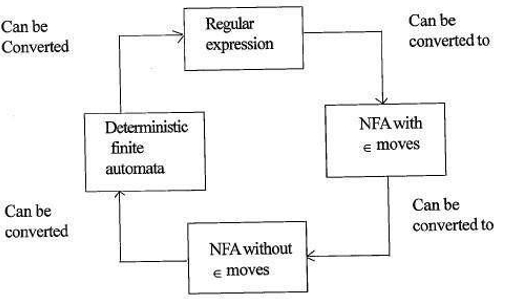# Explain the relationship between Finite Automata and Regular Expression.

In order to understand the relationship between finite automata (FA) and regular expression (RE), we need to understand these terminologies. Let us begin by understanding what is a regular expression.

## Regular Expression

Regular expression is the language which is used to describe the language and is accepted by finite automata. Regular expressions are the most effective way to represent any language. Let Σ be an alphabet which denotes the input set.

The regular expression over Σ can be defined as follows −

• Φ is a regular expression which denotes the empty set.
• ε is a regular expression and denotes the set { ε} and it is called a null string.
• For each ‘a’ in Σ ‘a’ is a regular expression and denotes the set {a}.
• If r and s regular expressions denoting the language.
• L1 and l2 respectively then,
• r+s is equivalent to L1 U L2 union
• rs is equivalent to L1L2 concatenation
• r* is equivalent to L1* closure

The r* is known as Kleen closure or closure which indicates occurrence of r for an infinite number of times.

Now, let us learn about finite automata.

## Finite Automata

Finite automata is an abstract computing device. It is a mathematical model of a system with discrete inputs, outputs, states and a set of transitions from state to state that occurs on input symbols from the alphabet Σ.

## Formal definition of Finite Automata

Finite automata is defined as a 5-tuples

M=(Q, Σ, δ,q0,F)

Where,

• Q: Finite set called states.
• Σ: Finite set called alphabets.
• δ: Q × Σ → Q is the transition function.
• q0 ∈ Q is the start or initial state.
• F: Final or accept state.

Now that we have learnt about these terminologies, let us understand the relation between them.

## Relationship

The relationship between FA and RE is as follows −The above figure explains that it is easy to convert

• RE to Non-deterministic finite automata (NFA) with epsilon moves.
• NFA with epsilon moves to without epsilon moves.
• NFA without epsilon moves to Deterministic Finite Automata (DFA).
• DFA can be converted easily to RE.